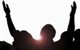# QlikView App Dev

Discussion Board for collaboration related to QlikView App Development.

Announcements
Customer & Partners, DEC. 9, 11 AM ET: Qlik Product & Strategy Roadmap Session: Data Analytics REGISTER NOW
cancel
Showing results for
Did you mean:MVP

## string matching with fuzzy, trigram (n-gram), levenshtein, etc.

Hi,

I'm looking for a possibility for string matching with fuzzy(-search), trigram (n-gram), levenshtein, etc. in QV script.

Any suggestions?

Ralf

26 RepliesMVP
Author

We've made an implementation of Metaphone and Kölner Phonetik (special for German: http://de.wikipedia.org/wiki/K%C3%B6lner_Phonetik) in our profiling solution TIQView with QV script.

You can use regex with VB Macro but this is not very stable for large datasets loaded with script. We also tested this out a lot...

- RalfMVP
Author

Another issue with VBScript regex is the limitation of regex operators! I hope for a plugable API to use Java or so in v10!

- RalfSpecialist

Ralf,

are you talking about a function in the VBScript in QV? like the one i posted for Levenshtein_distance?

something like:

function Levenshtein_distance

....

End functionMVP
Author

If you're asking for a regex VBScript Function, here we go:

Function regExpMatch(ByVal strVal, ByVal strPattern)
Set objRegExpr = New RegExp
objRegRegExpr.Pattern = "^" & strPattern & "\$"
objRegRegExpr.IgnoreCase = True
regExpMatch = objRegExpr.Test(strVal) * -1
End Function

- RalfSpecialist

i have something like that..

but those function cant be used in an expression right? only in load script?MVP
Author

..as far as I know, yes.

- RalfNot applicable

Levenshtein Distance :

 sub LevenshteinDistance(S1,S2)    let lns1 = len(S1);     let lns2 = len(S2);     for i=0 to \$(lns1)        let `d[\$(i),0]` = \$(i);        // trace d[\$(i),0] = \$(d[\$(i),0]);    next    for j=0 to \$(lns2)        let `d[0,\$(j)]` = \$(j);        // trace d[0,\$(j)] = \$(d[0,\$(j)]);    next    for j=1 to \$(lns2)        for i=1 to \$(lns1)                    let k = \$(i)-1;             let l = \$(j)-1;                // trace k : \$(k), trace l : \$(l);                let z = mid(S1,\$(i),1);            let w = mid(S2,\$(j),1);             // Trace z = \$(z) vs. w = \$(w);            if ('\$(z)'='\$(w)') then                 let `d[\$(i),\$(j)]` = \$(d[\$(k),\$(l)]);                // Trace `d[\$(i),\$(j)]` = \$(d[\$(i),\$(j)]);            else                let v1 = \$(d[\$(k),\$(j)]); // insertion                let v2 = \$(d[\$(i),\$(l)]); // deletion                let v3 = \$(d[\$(k),\$(l)]); // substitution                let `d[\$(i),\$(j)]` = rangemin(\$(v1),\$(v2),\$(v3))+1;                 // Trace `d[\$(i),\$(j)]` = \$(d[\$(i),\$(j)]);                end if                        next    next    let retour = \$(d[\$(lns1),\$(lns2)]);    Trace - word 1 : \$(S1);    Trace - word 2 : \$(S2);    Trace --------> retour = \$(retour) : \$(ids1s2) %;end subNot applicable

Hello,

Is it possible to see some code which implements the above?  I have this same requirement and its driving me crazy.  I have 2 files both with client names (they might be spelt differently e.g. Qlik ltd and Qlik Limited or Qliktech) and I need to present the end user with suggested matches.

Many thanksMVP
Author

Hi Karen,

I found a workable VBScript implementation as a function. This can be used during LOAD on record level. So you would need to join the source data first:

```Levenshtein:
LOAD F1, F2, levenshtein(F1,F2) as distance;
F1, F2
Qlik, Qlik ltd
Qlik ltd, Qlik Limited
Qlik Limited, QlikTech
Qlik, Klik
];

```

Module:

```' Source:
' http://en.wikibooks.org/wiki/Algorithm_Implementation/Strings/Levenshtein_distance#VBScript
Function levenshtein( a, b )
Dim i,j,cost,d,min1,min2,min3

' Avoid calculations where there there are empty words
If Len( a ) = 0 Then levenshtein = Len( b 😞 Exit Function
If Len( b ) = 0 Then levenshtein = Len( a 😞 Exit Function

' Array initialization
ReDim d( Len( a ), Len( b ) )

For i = 0 To Len( a 😞 d( i, 0 ) = i: Next
For j = 0 To Len( b 😞 d( 0, j ) = j: Next

' Actual calculation
For i = 1 To Len( a )
For j = 1 To Len( b )
If Mid(a, i, 1) = Mid(b, j, 1) Then cost = 0 Else cost = 1 End If

' Since min() function is not a part of VBScript, we'll "emulate" it below
min1 = ( d( i - 1, j ) + 1 )
min2 = ( d( i, j - 1 ) + 1 )
min3 = ( d( i - 1, j - 1 ) + cost )

If min1 <= min2 And min1 <= min3 Then
d( i, j ) = min1
ElseIf min2 <= min1 And min2 <= min3 Then
d( i, j ) = min2
Else
d( i, j ) = min3
End If
Next
Next

levenshtein = d( Len( a ), Len( b ) )
End Function
```

Hope this helps..

- Ralf